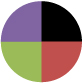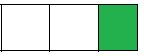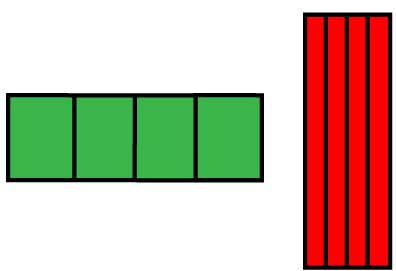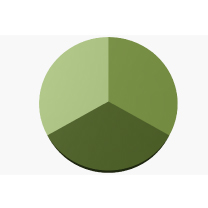Name:    2nd Grade Geometry - Reason with shapes and their attrributes, Standard 3,  Pre Test 1 Partition circles and rectangles into two, three, or four equal shares, describe the shares using the words halves, thirds, half of, a third of, etc., and describe the whole as two halves, three thirds, four fourths. Recognize that equal shares of identical wholes need not have the same shape.

Multiple Choice
Identify the choice that best completes the statement or answers the question.

1.Which word/phrase describes the part of the circle that is black?
 a. one c. one third b. one half d. one forth

2.Which word/phrase describes the part of the circle that is yellow?
 a. one c. one third b. one half d. one forth

3.Which word/phrase describes the part of the circle that is orange?
 a. one c. one third b. one half d. one forth

4.Which word/phrase describes the part of the rectangle that is green?
 a. a third c. a half b. a fourth d. one whole

5.Valrie saw the rectangle with one part colored green.  She said, “ _______ is green!”
 a. a third c. a half b. a fourth d. one whole

6.When Pete saw the circles, he said, “these circles are divided in ______!”
 a. fourths c. halves b. ones d. thirds

7.This rectangle has: ____________
 a. four fourths c. two halves b. ones whole d. three thirds

8.The circle on the left is divided the same as the circle on the right. They are both divided into _________
 a. fourths c. halves b. thirds d. ones

9.The retangle on the left is divided the same as the rectangle on the right. They are both divided into _________
 a. halves c. lines b. thirds d. fourths

Numeric Response

10.How many thirds does this circle have?

11.

If you draw a line right through the middle of a circle, how many halves do you get?